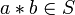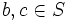# Tour:Associative binary operation

PREVIOUS: Invertible implies cancellative| UP: Introduction two (beginners)| NEXT: Inverse map is involutive
General instructions for the tour | Pedagogical notes for the tour | Pedagogical notes for this part
WHAT YOU NEED TO DO: Understand thoroughly the parenthesization can be dropped aspect of an associative binary operation (you don't need to work out the proof). This is crucial to manipulating expressions in groups, monoids, and semigroups.
PONDER: Try proving the statements about left, middle and right associative elements, to get a better understanding of how associativity works.

## Definition

### Definition in infix notation

Let$S$ be a set and$*$ be a binary operation on$S$ (viz,$*$ is a map$S \times S \to S$), making$(S,*)$ a magma. We denote$*$ using infix notation, so that its application to$x,y \in S$ is denoted$x * y$. Then,$*$ is said to be associative if, for every$a, b, c$ in$S$, the following identity holds:$(a * b) * c = a * (b * c)$

where equality holds as elements of$S$.

Note that$a,b,c$ are allowed to be equal or distinct. In particular, the above holds when all are equal, all are distinct, or two are equal and the third distinct.

Detailed explanation of expressions and their interpretation: The left side expression$(a * b) * c$ is termed the left associated expression for$a,b,c$ and is interpreted and evaluated as follows. We first consider$a * b$. Since$a,b \in S$, we have$a * b \in S$. We now consider the elements$a * b,c \in S$. Since both of these are in$S$,$(a * b) * c \in S$.

The right side expression$a * (b * c)$ is termed the right associated expression for$a,b,c$ and is interpreted and evaluated as follows. Since$b,c \in S$, we have$b * c \in S$. We consider consider the elements$a, b * c \in S$. Since both of these are in$S$,$a * (b * c) \in S$.

If, for a given$a, b, c$, the left associated expression and the right associated expression are equal,$a, b, c$ are said to associate. Associativity basically says that every ordered triple of elements associates.

### Definition in usual function notation

Let$S$ be a set and$f: S \times S \to S$ be a binary operation. We say that$f$ is associative if it satisfies the following for all$a,b,c \in S$:$f(f(a,b),c) = f(a,f(b,c))$

We see that the condition feels a lot less intuitive in function notation than with the infix notation, which is why infix notation is generally preferred for describing associativity in the context of binary operations.

### Related term

A set equipped with an associative binary operation is termed a semigroup. If, further, there is a neutral element (identity element) for the associative binary operation, the set is termed a monoid.

## Facts

### Parenthesization can be dropped

For full proof, refer: Associative implies generalized associative

When a binary operation is associative, it turns out that we can drop parenthesization from products of many elements. That is, given an expression of the form:$a_1 * a_2 ... * a_n$

any choice of bracketing will give the same result.

The result is proved by induction, with the base case ($n = 3$) following from the definition of associativity.

As an illustration, suppose we want to show that:$a_1 * (a_2 * (a_3 * a_4)) = ((a_1 * a_2) * a_3) * a_4$

Then, we apply associativity in a chain:$a_1 * (a_2 * (a_3 * a_4)) = a_1 * ((a_2 * a_3) * a_4) = (a_1 * (a_2 * a_3)) * a_4 = ((a_1 * a_2) * a_3) * a_4$

For this reason, we always use infix operator symbols for associative binary operations, and often even drop the operator symbol, so that the expression$a_1 * a_2 ... * a_n$ is just written as:$a_1a_2 \dots a_n$.

Also, the re-parenthesization identities (i.e., all identities that are special cases of generalized associativity) are the only identities that can be proved using associativity.

### Associativity pentagon

Further information: Associativity pentagon

The associativity pentagon is a pentagon whose vertices are the five different ways of associating a product of length four, with an edge between two vertices if moving from one to the other requires a single application of the associative law. This is a cyclic pentagon. The associativity pentagon is significant because, loosely, it generates all relations between the different ways of applying the associativity law to re-parenthesize expressions. It also helps to prove results about the set of left-associative, middle-associative, and right-associative elements. It is also related to the associator identity.

### Power structure

Further information: power-associative magma

In the presence of associativity, it is possible to unambiguously define positive powers of any element. Explicitly,$x^n$ is the$n$-fold product$x * x * \dots * x$. The powers satisfy the usual laws of powers:$x^m * x^n = x^{m + n}$ and$(x^m)^n = x^{mn}$ for all$m,n \in \mathbb{N}$. Note that this also implies that all powers of$x$ commute with each other.

Note that to define powers, we do not actually need global associativity, but only power-associativity: the submagma generated by any single element must be associative.